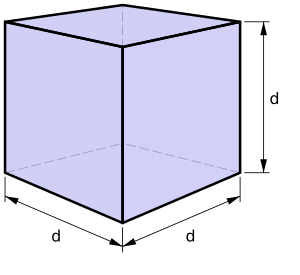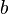# Base (geometry) facts for kids

Kids Encyclopedia FactsA cube is made of six squares; the square is therefore the base of the cube.

In plane geometry, the base is the side on which a polygon rests on, and which is used as a referenced side for other measurements. The base of a triangle or quadrilateral is often written as$b$. It is used in the calculation of the area of a triangle and parallelogram.

Many geometric shapes of more than two dimensions are constructed by using regular two-dimensional shapes. For example, a tetrahedron is constructed with four triangles, and a cube with six squares. In that context, the shape that is used for construction is called base. The triangle is the base of the tetrahedron, and the square is the base of the cube.

However, there are also geometric figures that are constructed using different shapes. A pyramid, for example. uses one rectangle and four triangles. In that context, the rectangle is said to be the base of the shape. A cylinder uses a circle or an ellipse as a base.

## Images for kidsBase (geometry) Facts for Kids. Kiddle Encyclopedia.